# Flow (continuous-time dynamical system)

(diff) ← Older revision | Latest revision (diff) | Newer revision → (diff)

A dynamical system determined by an action of the additive group of real numbers(or additive semi-group of non-negative real numbers) on a phase space. In other words, to each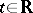(to each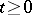) corresponds a transformation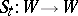such thatIn this caseis usually called "time" and the dependence ofon(for a fixed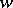) is said to be the "motion" of the point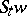; the set of allfor a givenis called the trajectory (or orbit) of(sometimes this term is used to describe the function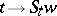). Just as for traditional dynamical systems the phase space of a flow usually is provided with a certain structure with which the flow is compatible: the transformations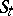preserve this structure and certain conditions are imposed on the manner in whichdepends on.

In applications one usually encounters flows described by autonomous systems (cf. Autonomous system) of ordinary differential equations(*)

or, in vector notation,,. The immediate generalization of a flow is a flow on a differentiable manifold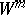defined ( "generated" ) by a smooth vector fieldof class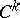,(a smooth flow of class) given on. In this case the motion of a point, as long as it stays within one chart (local coordinate system), is described by a system of the form (*), in the right-hand side of which one finds the components of the vector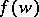in the corresponding coordinates. When passing to another chart the description of the motion changes, since in this case both the coordinates of the pointchange as well as the expressions for the components ofas functions of the local coordinates. See also Measurable flow; Continuous flow; Topological dynamical system.

Flows form the most important class of dynamical systems and were, moreover, the first to be studied. The term "dynamical system" is often used in a narrow sense, meaning precisely a flow (or a flow and a cascade).